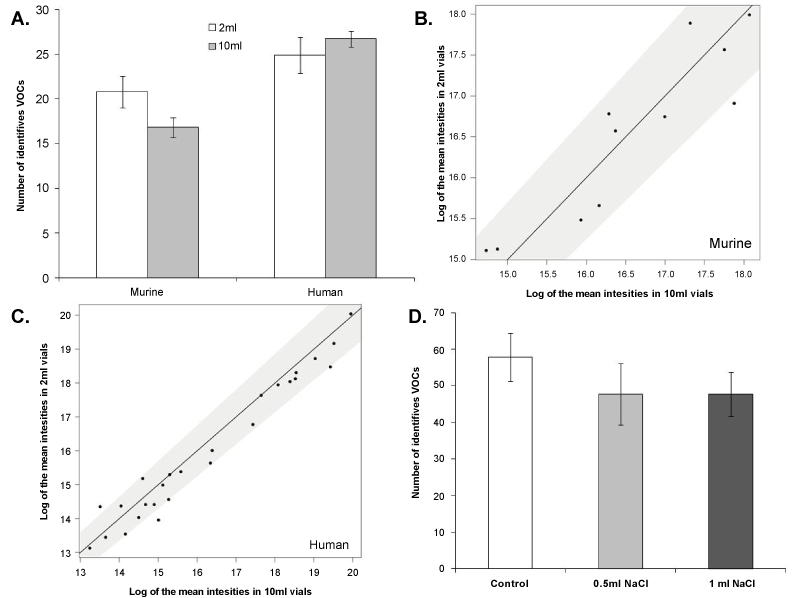Figure 3: Vial volume optimisation and salting-out. (A) Faecal samples collected from murine (n=4/volume, N=3) and human (n=4/volume; 10 ml=102.8 ± 1.7 mg, 2 ml=101.9 ± 1.2 mg, N=3) using two different vial volumes, 2 ml and 10 ml (mean ± SEM; t-test). (B-C) Comparison of the two vial volumes studied, showing the mean of the abundance for each identified compounds (murine=11, humans=26) per size of vial (n=4/time) for both murine (B) and human samples (C), plotted against the other. The grey area indicates the 5% tolerance around the optimal function x=y. (D) Faecal samples collected from an individual human treated with 0.5 ml NaCl and 1 ml NaCl and compared to a control sample (n=3/treatment; control=97.9 ± 2 mg, 0.5 ml NaCl=100.4 ± 3.6 mg, 1 ml NaCl=99.5 ± 3.2 mg) (mean ± SEM); ANOVA with Tukeys HSD followed by Bonferroni; *p<0.05, **p<0.01 and ***p<0.001.
Goto home»